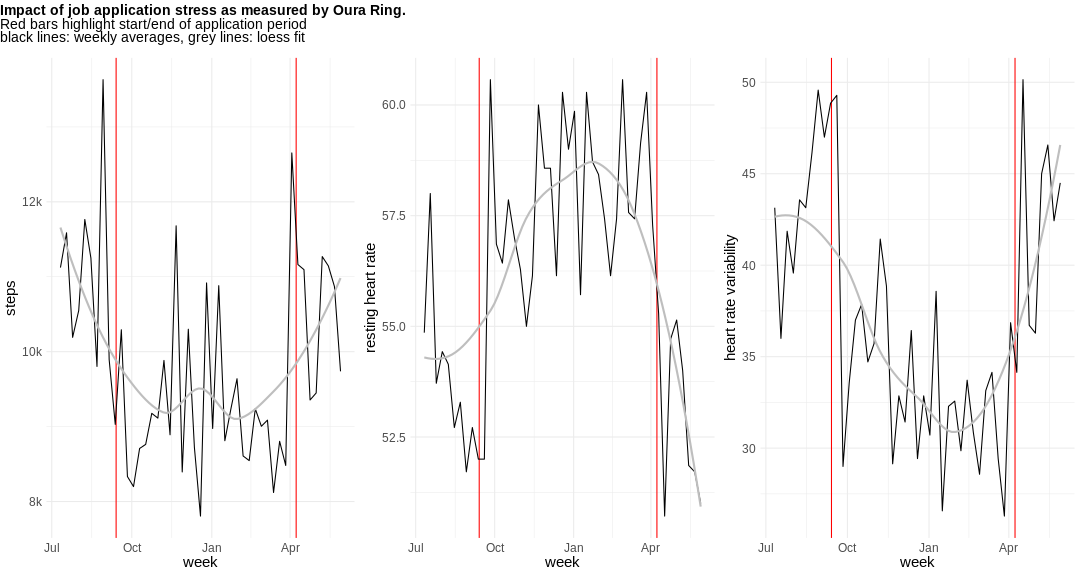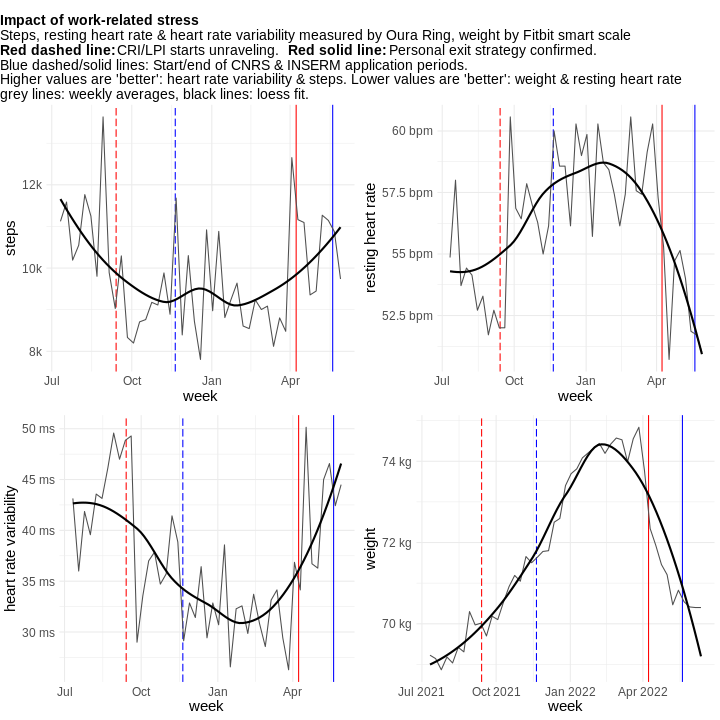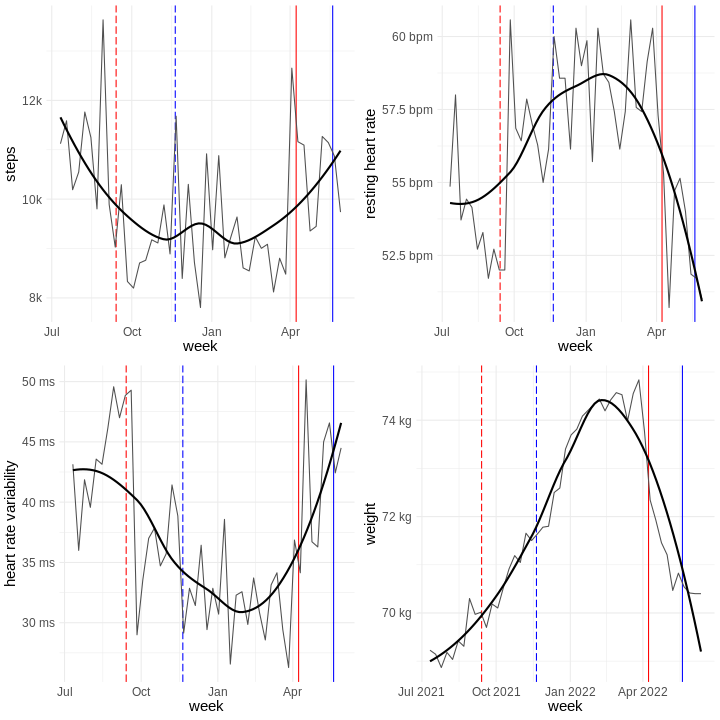# Details for `workstress-impact-analysis.ipynb`

#### Description

Looking into the impact of long-term occupational stress over time.

#### Tags & Data Sources

stress HRV heart rate Oura Connect Fitbit connection

# Work-related stress and its impact¶

If you want to run this notebook and run into problems or have questions: Reach out to Bastian on Twitter or Slack

This notebook was used to create the self-research project Impact of work-related stress. It uses data from an Oura Ring and a Fitbit smart scale. If you want to run this analysis for your data you need the following data sources connected to your Open Humans account (or selectively run it):

## Plotting the effects¶

There will be some inherent noise in day-to-day measures as behaviour changes depending on the day of the week (just think of weekdays vs weekends) To remove a good bit of this variance I'm taking only the weekly mean values (in case of sleep/activity) or the sum of all values within a week (in case of productivity).

```/opt/conda/lib/python3.7/site-packages/rpy2/robjects/pandas2ri.py:14: FutureWarning: pandas.core.index is deprecated and will be removed in a future version.  The public classes are available in the top-level namespace.
from pandas.core.index import Index as PandasIndex
```
```WARNING:rpy2.rinterface_lib.callbacks:R[write to console]:
Attaching package: ‘lubridate’

WARNING:rpy2.rinterface_lib.callbacks:R[write to console]: The following objects are masked from ‘package:base’:

date, intersect, setdiff, union

WARNING:rpy2.rinterface_lib.callbacks:R[write to console]:
Attaching package: ‘cowplot’

WARNING:rpy2.rinterface_lib.callbacks:R[write to console]: The following object is masked from ‘package:lubridate’:

stamp

```

Warning: These widgets currently don't work in the Voila display as they use a hacky Javascript solution to re-run the notebook. If you want to edit those values you unfortunately have to do so from the regular Jupyter Notebook interface

# Effect on HR & HRV (Oura)¶```WARNING:rpy2.rinterface_lib.callbacks:R[write to console]: trying URL 'http://cran.us.r-project.org/src/contrib/ggtext_0.1.1.tar.gz'

WARNING:rpy2.rinterface_lib.callbacks:R[write to console]: Content type 'application/x-gzip'
WARNING:rpy2.rinterface_lib.callbacks:R[write to console]:  length 1211846 bytes (1.2 MB)

WARNING:rpy2.rinterface_lib.callbacks:R[write to console]: =
WARNING:rpy2.rinterface_lib.callbacks:R[write to console]: =
WARNING:rpy2.rinterface_lib.callbacks:R[write to console]: =
WARNING:rpy2.rinterface_lib.callbacks:R[write to console]: =
WARNING:rpy2.rinterface_lib.callbacks:R[write to console]: =
WARNING:rpy2.rinterface_lib.callbacks:R[write to console]: =
WARNING:rpy2.rinterface_lib.callbacks:R[write to console]: =
WARNING:rpy2.rinterface_lib.callbacks:R[write to console]: =
WARNING:rpy2.rinterface_lib.callbacks:R[write to console]: =
WARNING:rpy2.rinterface_lib.callbacks:R[write to console]: =
WARNING:rpy2.rinterface_lib.callbacks:R[write to console]: =
WARNING:rpy2.rinterface_lib.callbacks:R[write to console]: =
WARNING:rpy2.rinterface_lib.callbacks:R[write to console]: =
WARNING:rpy2.rinterface_lib.callbacks:R[write to console]: =
WARNING:rpy2.rinterface_lib.callbacks:R[write to console]: =
WARNING:rpy2.rinterface_lib.callbacks:R[write to console]: =
WARNING:rpy2.rinterface_lib.callbacks:R[write to console]: =
WARNING:rpy2.rinterface_lib.callbacks:R[write to console]: =
WARNING:rpy2.rinterface_lib.callbacks:R[write to console]: =
WARNING:rpy2.rinterface_lib.callbacks:R[write to console]: =
WARNING:rpy2.rinterface_lib.callbacks:R[write to console]: =
WARNING:rpy2.rinterface_lib.callbacks:R[write to console]: =
WARNING:rpy2.rinterface_lib.callbacks:R[write to console]: =
WARNING:rpy2.rinterface_lib.callbacks:R[write to console]: =
WARNING:rpy2.rinterface_lib.callbacks:R[write to console]: =
WARNING:rpy2.rinterface_lib.callbacks:R[write to console]: =
WARNING:rpy2.rinterface_lib.callbacks:R[write to console]: =
WARNING:rpy2.rinterface_lib.callbacks:R[write to console]: =
WARNING:rpy2.rinterface_lib.callbacks:R[write to console]: =
WARNING:rpy2.rinterface_lib.callbacks:R[write to console]: =
WARNING:rpy2.rinterface_lib.callbacks:R[write to console]: =
WARNING:rpy2.rinterface_lib.callbacks:R[write to console]: =
WARNING:rpy2.rinterface_lib.callbacks:R[write to console]: =
WARNING:rpy2.rinterface_lib.callbacks:R[write to console]: =
WARNING:rpy2.rinterface_lib.callbacks:R[write to console]: =
WARNING:rpy2.rinterface_lib.callbacks:R[write to console]: =
WARNING:rpy2.rinterface_lib.callbacks:R[write to console]: =
WARNING:rpy2.rinterface_lib.callbacks:R[write to console]: =
WARNING:rpy2.rinterface_lib.callbacks:R[write to console]: =
WARNING:rpy2.rinterface_lib.callbacks:R[write to console]: =
WARNING:rpy2.rinterface_lib.callbacks:R[write to console]: =
WARNING:rpy2.rinterface_lib.callbacks:R[write to console]: =
WARNING:rpy2.rinterface_lib.callbacks:R[write to console]: =
WARNING:rpy2.rinterface_lib.callbacks:R[write to console]: =
WARNING:rpy2.rinterface_lib.callbacks:R[write to console]: =
WARNING:rpy2.rinterface_lib.callbacks:R[write to console]: =
WARNING:rpy2.rinterface_lib.callbacks:R[write to console]: =
WARNING:rpy2.rinterface_lib.callbacks:R[write to console]: =
WARNING:rpy2.rinterface_lib.callbacks:R[write to console]: =
WARNING:rpy2.rinterface_lib.callbacks:R[write to console]: =
WARNING:rpy2.rinterface_lib.callbacks:R[write to console]:

WARNING:rpy2.rinterface_lib.callbacks:R[write to console]:

WARNING:rpy2.rinterface_lib.callbacks:R[write to console]:
WARNING:rpy2.rinterface_lib.callbacks:R[write to console]:
WARNING:rpy2.rinterface_lib.callbacks:R[write to console]:

WARNING:rpy2.rinterface_lib.callbacks:R[write to console]: Updating HTML index of packages in '.Library'

WARNING:rpy2.rinterface_lib.callbacks:R[write to console]: Making 'packages.html' ...
WARNING:rpy2.rinterface_lib.callbacks:R[write to console]:  done

```

# Plot everything with caption¶# Plot without caption¶# Work-related stress and its impact¶

If you want to run this notebook and run into problems or have questions: Reach out to Bastian on Twitter or Slack

This notebook was used to create the self-research project Impact of work-related stress. It uses data from an Oura Ring and a Fitbit smart scale. If you want to run this analysis for your data you need the following data sources connected to your Open Humans account (or selectively run it):

In :
```### GET DATA FOR RESCUETIME, OURA AND SPOTIFY

from ohapi import api
import os
import requests
import tempfile
import json
import pandas as pd
from datetime import datetime

oura_present = ""
rescuetime_present = ""
spotify_present = ""

df_moment = ""
dataframe_oura_full = ""
rt_df_full = ""
df_spotify = ""
overland_subset = ""

user_details = api.exchange_oauth2_member(os.environ.get('OH_ACCESS_TOKEN'))
for i in user_details['data']:
if i['source'] == 'direct-sharing-184' and i['basename'] == 'oura-data.json':
oura_present = "True"
```
In :
```### PARSERS FOR OURA, SPOTIFY & RESCUETIME

dates = []
values = []
value_type = []

for sdate in oura['sleep']:
dates.append(sdate['summary_date'])
if 'score' in sdate.keys():
values.append(sdate['score'])
else:
values.append(0)
value_type.append('sleep')
dates.append(sdate['summary_date'])
values.append(sdate['total'])
value_type.append('sleep_sum')

dates.append(sdate['summary_date'])
values.append(sdate['hr_lowest'])
value_type.append('hr_lowest')

dates.append(sdate['summary_date'])
values.append(sdate['rmssd'])
value_type.append('hrv')

for sdate in oura['activity']:
dates.append(sdate['summary_date'])
if 'score' in sdate.keys():
values.append(sdate['score'])
else:
values.append(0)
value_type.append('activity')
dates.append(sdate['summary_date'])
values.append(sdate['steps'])
value_type.append('steps')

dates.append(sdate['summary_date'])
values.append(sdate['score'])

dataframe = pd.DataFrame(
data = {
'date': dates,
'value': values,
'type': value_type
}
)
return dataframe

def parse_timestamp(lst):
timestamps = []
for item in lst:
try:
timestamp = datetime.strptime(
item,
'%Y-%m-%dT%H:%M:%S.%fZ')
except ValueError:
timestamp = datetime.strptime(
item,
'%Y-%m-%dT%H:%M:%SZ')
timestamps.append(timestamp)
return timestamps
```
In :
```### CREATE DATAFRAMES
if oura_present:
```

## Plotting the effects¶

There will be some inherent noise in day-to-day measures as behaviour changes depending on the day of the week (just think of weekdays vs weekends) To remove a good bit of this variance I'm taking only the weekly mean values (in case of sleep/activity) or the sum of all values within a week (in case of productivity).

In :
```%load_ext rpy2.ipython
```
```/opt/conda/lib/python3.7/site-packages/rpy2/robjects/pandas2ri.py:14: FutureWarning: pandas.core.index is deprecated and will be removed in a future version.  The public classes are available in the top-level namespace.
from pandas.core.index import Index as PandasIndex
```
In :
```%%R -i dataframe_oura_full,oura_present -w 10 -h 10 --units in

## here we load the R packages and submit our processed data to the R kernel which will take care of all the rest
library(lubridate)
library(ggplot2)
if (!'cowplot' %in% installed.packages()) install.packages('cowplot',repos = "http://cran.us.r-project.org")
library(cowplot)
if (oura_present != ""){
dataframe_oura_full\$date <- as.Date(dataframe_oura_full\$date)
dataframe_oura_full\$week <- floor_date(dataframe_oura_full\$date,unit='week')
df_oura_agg_full <- aggregate(value~week+type,data=dataframe_oura_full,FUN=mean)
}
```
```WARNING:rpy2.rinterface_lib.callbacks:R[write to console]:
Attaching package: ‘lubridate’

WARNING:rpy2.rinterface_lib.callbacks:R[write to console]: The following objects are masked from ‘package:base’:

date, intersect, setdiff, union

WARNING:rpy2.rinterface_lib.callbacks:R[write to console]:
Attaching package: ‘cowplot’

WARNING:rpy2.rinterface_lib.callbacks:R[write to console]: The following object is masked from ‘package:lubridate’:

stamp

```
In :
```from IPython.display import Javascript, display
from ipywidgets import widgets
import datetime

def run_all(ev):
display(Javascript('IPython.notebook.execute_cells_below()'))

button = widgets.Button(description="Update plots!")

ld_start = widgets.DatePicker(
description='Start',
disabled=False,
value=datetime.datetime(2021,9,13).date()
)

ld_end = widgets.DatePicker(
description='End',
disabled=False,
value=datetime.datetime(2022,4,8).date()
)

boundaries = widgets.IntSlider(
value=10,
min=2,
max=20,
step=1,
description='Weeks before/after lockdown:',
disabled=False,
orientation='horizontal',
)

button.on_click(run_all)
display(ld_start,ld_end,boundaries)
```
In :
```display(button)
```

Warning: These widgets currently don't work in the Voila display as they use a hacky Javascript solution to re-run the notebook. If you want to edit those values you unfortunately have to do so from the regular Jupyter Notebook interface

In :
```START_DATE = str(ld_start.value)
END_DATE = str(ld_end.value)
WEEKS_BOUNDING = boundaries.value
```

# Effect on HR & HRV (Oura)¶

In :
```%%R  -w 15 -h 8 --units in -i START_DATE,END_DATE,WEEKS_BOUNDING
if (oura_present != ""){
step_plot <- ggplot(subset(df_oura_agg_full, df_oura_agg_full\$week > as.Date(START_DATE) - weeks(WEEKS_BOUNDING) & df_oura_agg_full\$week < as.Date(END_DATE) + weeks(WEEKS_BOUNDING) & as.character(df_oura_agg_full\$type) %in% c('steps')), aes(x=week,y=value/1000)) +
geom_vline(xintercept=as.Date(START_DATE), color='red') +
geom_vline(xintercept=as.Date(END_DATE), color='red') +
geom_line() + theme_minimal() +
geom_smooth(se = FALSE,color='grey',method='loess',formula='y ~ x') +
scale_y_continuous("steps",labels = function(x) paste0(x, "k")) +
labs(
) + theme(text = element_text(size=15)) +
theme(plot.caption= element_text(size=9))

hr_plot <- ggplot(subset(df_oura_agg_full, df_oura_agg_full\$week > as.Date(START_DATE) - weeks(WEEKS_BOUNDING) & df_oura_agg_full\$week < as.Date(END_DATE) + weeks(WEEKS_BOUNDING) & as.character(df_oura_agg_full\$type) %in% c('hr_lowest')), aes(x=week,y=value)) +
geom_vline(xintercept=as.Date(START_DATE), color='red') +
geom_vline(xintercept=as.Date(END_DATE), color='red') +
geom_line() + theme_minimal() +
geom_smooth(se = FALSE,color='grey',method='loess',formula='y ~ x') +
scale_y_continuous("resting heart rate") +
labs(
) + theme(text = element_text(size=15)) +
theme(plot.caption= element_text(size=9))

hrv_plot <- ggplot(subset(df_oura_agg_full, df_oura_agg_full\$week > as.Date(START_DATE) - weeks(WEEKS_BOUNDING) & df_oura_agg_full\$week < as.Date(END_DATE) + weeks(WEEKS_BOUNDING) & as.character(df_oura_agg_full\$type) %in% c('hrv')), aes(x=week,y=value)) +
geom_vline(xintercept=as.Date(START_DATE), color='red') +
geom_vline(xintercept=as.Date(END_DATE), color='red') +
geom_line() + theme_minimal() +
geom_smooth(se = FALSE,color='grey',method='loess',formula='y ~ x') +
scale_y_continuous("heart rate variability") +
labs(
) + theme(text = element_text(size=15)) +
theme(plot.caption= element_text(size=9))

title <- ggdraw() +
draw_label(
"Impact of job application stress as measured by Oura Ring.",
fontface = 'bold',
x = 0,
y= 0.8,
hjust = 0
) +
draw_label(
"Red bars highlight start/end of application period",
x = 0,
y = 0.55,
hjust = 0
)+
draw_label(
"black lines: weekly averages, grey lines: loess fit",
x = 0,
y = 0.3,
hjust = 0
)

plot_grid(title,plot_grid(step_plot,hr_plot,hrv_plot, ncol=3,  rel_heights = c(1,1,1)),nrow=2,rel_heights=c(0.1,1))
}
```In :
```%%R
install.packages("ggtext", repos = "http://cran.us.r-project.org")
library(ggtext)
```
```WARNING:rpy2.rinterface_lib.callbacks:R[write to console]: trying URL 'http://cran.us.r-project.org/src/contrib/ggtext_0.1.1.tar.gz'

WARNING:rpy2.rinterface_lib.callbacks:R[write to console]: Content type 'application/x-gzip'
WARNING:rpy2.rinterface_lib.callbacks:R[write to console]:  length 1211846 bytes (1.2 MB)

WARNING:rpy2.rinterface_lib.callbacks:R[write to console]: =
WARNING:rpy2.rinterface_lib.callbacks:R[write to console]: =
WARNING:rpy2.rinterface_lib.callbacks:R[write to console]: =
WARNING:rpy2.rinterface_lib.callbacks:R[write to console]: =
WARNING:rpy2.rinterface_lib.callbacks:R[write to console]: =
WARNING:rpy2.rinterface_lib.callbacks:R[write to console]: =
WARNING:rpy2.rinterface_lib.callbacks:R[write to console]: =
WARNING:rpy2.rinterface_lib.callbacks:R[write to console]: =
WARNING:rpy2.rinterface_lib.callbacks:R[write to console]: =
WARNING:rpy2.rinterface_lib.callbacks:R[write to console]: =
WARNING:rpy2.rinterface_lib.callbacks:R[write to console]: =
WARNING:rpy2.rinterface_lib.callbacks:R[write to console]: =
WARNING:rpy2.rinterface_lib.callbacks:R[write to console]: =
WARNING:rpy2.rinterface_lib.callbacks:R[write to console]: =
WARNING:rpy2.rinterface_lib.callbacks:R[write to console]: =
WARNING:rpy2.rinterface_lib.callbacks:R[write to console]: =
WARNING:rpy2.rinterface_lib.callbacks:R[write to console]: =
WARNING:rpy2.rinterface_lib.callbacks:R[write to console]: =
WARNING:rpy2.rinterface_lib.callbacks:R[write to console]: =
WARNING:rpy2.rinterface_lib.callbacks:R[write to console]: =
WARNING:rpy2.rinterface_lib.callbacks:R[write to console]: =
WARNING:rpy2.rinterface_lib.callbacks:R[write to console]: =
WARNING:rpy2.rinterface_lib.callbacks:R[write to console]: =
WARNING:rpy2.rinterface_lib.callbacks:R[write to console]: =
WARNING:rpy2.rinterface_lib.callbacks:R[write to console]: =
WARNING:rpy2.rinterface_lib.callbacks:R[write to console]: =
WARNING:rpy2.rinterface_lib.callbacks:R[write to console]: =
WARNING:rpy2.rinterface_lib.callbacks:R[write to console]: =
WARNING:rpy2.rinterface_lib.callbacks:R[write to console]: =
WARNING:rpy2.rinterface_lib.callbacks:R[write to console]: =
WARNING:rpy2.rinterface_lib.callbacks:R[write to console]: =
WARNING:rpy2.rinterface_lib.callbacks:R[write to console]: =
WARNING:rpy2.rinterface_lib.callbacks:R[write to console]: =
WARNING:rpy2.rinterface_lib.callbacks:R[write to console]: =
WARNING:rpy2.rinterface_lib.callbacks:R[write to console]: =
WARNING:rpy2.rinterface_lib.callbacks:R[write to console]: =
WARNING:rpy2.rinterface_lib.callbacks:R[write to console]: =
WARNING:rpy2.rinterface_lib.callbacks:R[write to console]: =
WARNING:rpy2.rinterface_lib.callbacks:R[write to console]: =
WARNING:rpy2.rinterface_lib.callbacks:R[write to console]: =
WARNING:rpy2.rinterface_lib.callbacks:R[write to console]: =
WARNING:rpy2.rinterface_lib.callbacks:R[write to console]: =
WARNING:rpy2.rinterface_lib.callbacks:R[write to console]: =
WARNING:rpy2.rinterface_lib.callbacks:R[write to console]: =
WARNING:rpy2.rinterface_lib.callbacks:R[write to console]: =
WARNING:rpy2.rinterface_lib.callbacks:R[write to console]: =
WARNING:rpy2.rinterface_lib.callbacks:R[write to console]: =
WARNING:rpy2.rinterface_lib.callbacks:R[write to console]: =
WARNING:rpy2.rinterface_lib.callbacks:R[write to console]: =
WARNING:rpy2.rinterface_lib.callbacks:R[write to console]: =
WARNING:rpy2.rinterface_lib.callbacks:R[write to console]:

WARNING:rpy2.rinterface_lib.callbacks:R[write to console]:

WARNING:rpy2.rinterface_lib.callbacks:R[write to console]:
WARNING:rpy2.rinterface_lib.callbacks:R[write to console]:
WARNING:rpy2.rinterface_lib.callbacks:R[write to console]:

WARNING:rpy2.rinterface_lib.callbacks:R[write to console]: Updating HTML index of packages in '.Library'

WARNING:rpy2.rinterface_lib.callbacks:R[write to console]: Making 'packages.html' ...
WARNING:rpy2.rinterface_lib.callbacks:R[write to console]:  done

```

# Impact on weight¶

In :
```dates = []
weights = []

user_details = api.exchange_oauth2_member(os.environ.get('OH_ACCESS_TOKEN'))
for i in user_details['data']:
if i['basename'] == 'fitbit-data.json':
for year in fitbit['weight'].keys():
for element in fitbit['weight'][year]['body-weight']:
dates.append(element['dateTime'])
weights.append(element['value'])

weight_df = pd.DataFrame(data={
'date': dates,
'weight': weights
})
```
In :
```%%R -i weight_df -w 10 -h 10 --units in

weight_df\$date <- as.Date(weight_df\$date)
weight_df\$week <- floor_date(weight_df\$date,unit='week')

weight_df\$weight <- as.numeric(weight_df\$weight)

weight_agg <- aggregate(weight~week,data=weight_df,FUN=mean)
```
In :
```%%R -i weight_df -w 10 -h 10 --units in

weight_df\$date <- as.Date(weight_df\$date)

weight_df\$weight <- as.numeric(weight_df\$weight)

weight_plot <- ggplot(subset(weight_agg, weight_agg\$week > as.Date(START_DATE) - weeks(WEEKS_BOUNDING) & weight_agg\$week < as.Date(END_DATE) + weeks(WEEKS_BOUNDING)), aes(x=week,y=weight)) +
geom_vline(xintercept=as.Date('2021-11-20'), color='blue',linetype='longdash') +
geom_vline(xintercept=as.Date('2022-05-20'), color='blue') +
geom_vline(xintercept=as.Date(START_DATE), color='red', linetype='longdash') +
geom_vline(xintercept=as.Date(END_DATE), color='red') +
geom_line(color='gray31') + theme_minimal() +
geom_smooth(se = FALSE,color='black',method='loess',formula='y ~ x') +
scale_y_continuous("weight",labels = function(x) paste0(x, " kg")) +
labs(
) + theme(text = element_text(size=15)) +
theme(plot.caption= element_text(size=9))
```

# Plot everything with caption¶

In :
```%%R  -w 10 -h 10 --units in
if (oura_present != ""){
step_plot <- ggplot(subset(df_oura_agg_full, df_oura_agg_full\$week > as.Date(START_DATE) - weeks(WEEKS_BOUNDING) & df_oura_agg_full\$week < as.Date(END_DATE) + weeks(WEEKS_BOUNDING) & as.character(df_oura_agg_full\$type) %in% c('steps')), aes(x=week,y=value/1000)) +
geom_vline(xintercept=as.Date('2021-11-20'), color='blue',linetype='longdash') +
geom_vline(xintercept=as.Date('2022-05-20'), color='blue') +
geom_vline(xintercept=as.Date(START_DATE), color='red',linetype='longdash') +
geom_vline(xintercept=as.Date(END_DATE), color='red') +
geom_line(color='gray31') + theme_minimal() +
geom_smooth(se = FALSE,color='black',method='loess',formula='y ~ x') +
scale_y_continuous("steps",labels = function(x) paste0(x, "k")) +
labs(
) + theme(text = element_text(size=15)) +
theme(plot.caption= element_text(size=9))

hr_plot <- ggplot(subset(df_oura_agg_full, df_oura_agg_full\$week > as.Date(START_DATE) - weeks(WEEKS_BOUNDING) & df_oura_agg_full\$week < as.Date(END_DATE) + weeks(WEEKS_BOUNDING) & as.character(df_oura_agg_full\$type) %in% c('hr_lowest')), aes(x=week,y=value)) +
geom_vline(xintercept=as.Date('2021-11-20'), color='blue',linetype='longdash') +
geom_vline(xintercept=as.Date('2022-05-20'), color='blue') +
geom_vline(xintercept=as.Date(START_DATE), color='red', linetype='longdash') +
geom_vline(xintercept=as.Date(END_DATE), color='red') +
geom_line(color='gray31') + theme_minimal() +
geom_smooth(se = FALSE,color='black',method='loess',formula='y ~ x') +
scale_y_continuous("resting heart rate",labels = function(x) paste0(x, " bpm")) +
labs(
) + theme(text = element_text(size=15)) +
theme(plot.caption= element_text(size=9))

hrv_plot <- ggplot(subset(df_oura_agg_full, df_oura_agg_full\$week > as.Date(START_DATE) - weeks(WEEKS_BOUNDING) & df_oura_agg_full\$week < as.Date(END_DATE) + weeks(WEEKS_BOUNDING) & as.character(df_oura_agg_full\$type) %in% c('hrv')), aes(x=week,y=value)) +
geom_vline(xintercept=as.Date('2021-11-20'), color='blue',linetype='longdash') +
geom_vline(xintercept=as.Date('2022-05-20'), color='blue') +
geom_vline(xintercept=as.Date(START_DATE), color='red', linetype='longdash') +
geom_vline(xintercept=as.Date(END_DATE), color='red') +
geom_line(color='gray31') + theme_minimal() +
geom_smooth(se = FALSE,color='black',method='loess',formula='y ~ x') +
scale_y_continuous("heart rate variability", ,labels = function(x) paste0(x, " ms")) +
labs(
) + theme(text = element_text(size=15)) +
theme(plot.caption= element_text(size=9))

title <- ggdraw() +
draw_label(
"Impact of work-related stress",
fontface = 'bold',
x = 0,
y= 0.8,
hjust = 0
) +
draw_label(
"Steps, resting heart rate & heart rate variability measured by Oura Ring, weight by Fitbit smart scale",
x = 0,
y = 0.65,
hjust = 0
)+
draw_label(
"Red dashed line:",
x = 0,
y = 0.5,
hjust = 0,
fontface='bold'
)+
draw_label(
"CRI/LPI starts unraveling.",
x = 0.162,
y = 0.5,
hjust = 0
)+
draw_label(
"Red solid line:",
x = 0.4,
y = 0.5,
hjust = 0,
fontface='bold'
)+
draw_label(
"Personal exit strategy confirmed.",
x = 0.54,
y = 0.5,
hjust = 0
)+
draw_label(
"Blue dashed/solid lines: Start/end of CNRS & INSERM application periods.",
x = 0,
y = 0.35,
hjust = 0
) +
draw_label(
"Higher values are 'better': heart rate variability & steps. Lower values are 'better': weight & resting heart rate",
x = 0,
y = 0.2,
hjust = 0
) +
draw_label(
"grey lines: weekly averages, black lines: loess fit.",
x = 0,
y = 0.05,
hjust = 0
)

plot_grid(title,plot_grid(step_plot,hr_plot,hrv_plot, weight_plot, ncol=2,  rel_heights = c(1,1,1)),nrow=2,rel_heights=c(0.16,1))
}
```# Plot without caption¶

In :
```%%R -w 10 -h 10 --units in

plot_grid(step_plot,hr_plot,hrv_plot, weight_plot, ncol=2,  rel_heights = c(1,1,1))
```